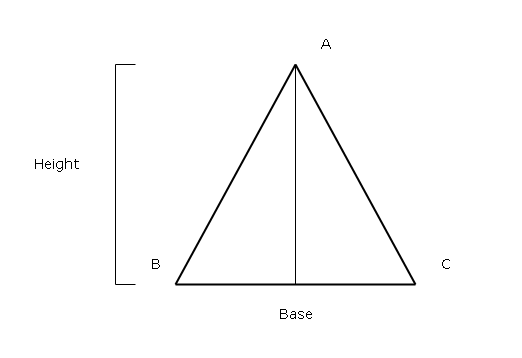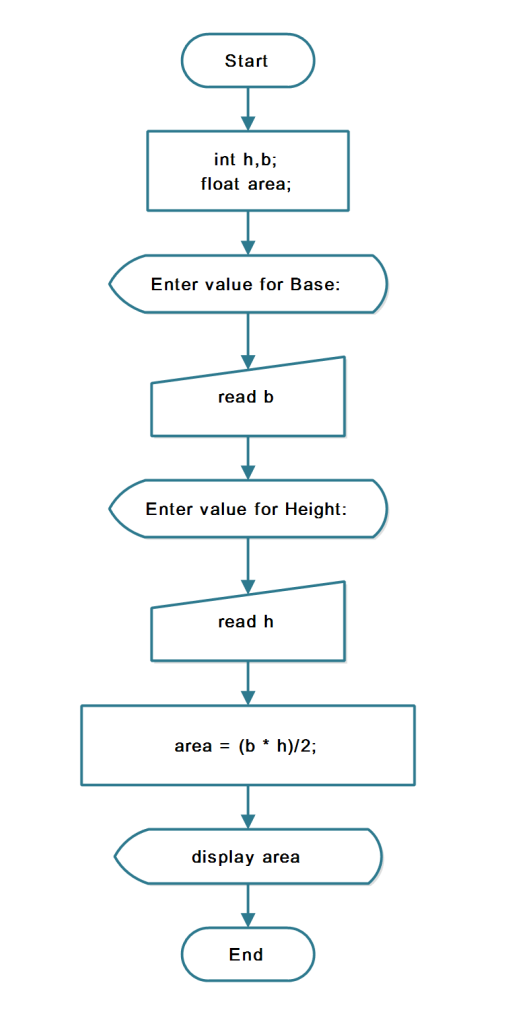## Program to find Area of a Triangle in Java

The program to find the area of a triangle is a simple program that demonstrates the program structure of Java. It is intended for beginner level learner of Java programming.

Also, we used NetBean 8.2 on a windows 7 64-bit with Java SDK 8u111 to write this program.

To help you understand the program read following sections – problem definition, flowchart, program code for practice and verified output.

## Problem Definition

A triangle is a geometric shape with 3 sides and sum of internal angles make 180 degrees.The following figure shows a triangle with three sides.Figure 1 – Triangle with Base and Height

To find the area of a triangle you need to know the length of base and the length of height and use the following formula.
``````Area of Triangle = base * (height /2)

Or

Area of Triangle = b * (h/2)
``````

## FlowchartFlowchart: Java Program to find Area of a Triangle

## Program Code

``````/*
* To change this template file, choose Tools | Templates
* and open the template in the editor.
*/

package JavaExamples;
import java.io.*;
/**
*
*/
public class AreaTriangle {

public static void main(String args[])
{

int b, h;
float area;

try {

DataInputStream d = new DataInputStream((System.in));

System.out.println("Enter value for Base:");

System.out.println("Enter value for Height:");

b = Integer.parseInt(st);

h = Integer.parseInt(sh);

System.out.println("b=" + b);

System.out.println("h=" + h);

area = (b * h) / 2;

System.out.println("Area of triangle=" + area);

} catch (IOException e)   {

System.out.println("Wrong Input");

}

}

}

``````

## Output

``````Enter value for Base:

20

Enter value for Height:

12

b=20

h=12

Area of triangle= 120.0``````

Related Articles:-